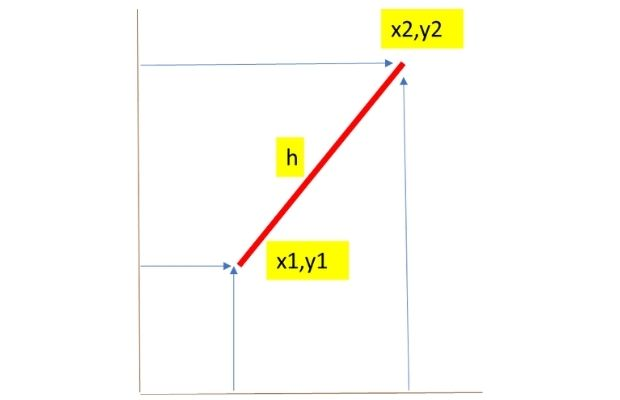# sqrt()

sqrt(x) returns squqre root of input number as float data type.
``````import math
print(math.sqrt(4))    # 2.0
print(math.sqrt(16))   # 4.0
print(math.sqrt(20))   # 4.47213595499958
print(math.sqrt(0))    # 0.0``````
Using negative number
For any value less than 0 , we will get ValueError
``````import math
print(math.sqrt(-15)) ``````
Above code will generate error.

Output of math.sqrt() is float data type. We can use type() to get the data type.
``````import math
x=math.sqrt(25)
print(x)        # 5.0
print(type(x))  # <class 'float'>``````
We can use int() to convert output to integer data type.
``````import math
x=math.sqrt(25)
y=int(x)
print(type(y))  # <class 'int'>``````

## Length from X Y coordinatesIf X and Y coordinates of starting and ending points of a line is given then the length can be calculated.
``````x1=13639.4
y1=1554.8
x2=13637.5
y2=1570.6
import math
h=math.sqrt((x2-x1)**2 + (y2-y1)**2)
print(h)``````
We will use round() to limit our output to 2 decimal places.
``h=round(math.sqrt((x2-x1)**2 + (y2-y1)**2),2) ``
Assuming that the input coordinates are in meter , we can get the output in feet and Kadi.
``````in_feet=h*3.2808
print("Length meeter : ",round(h,2)," Feet:",round(in_feet,2),"
Conversion formula is here
``````1 Feet = 0.3048 Meter
1 Meter = 3.2808 Feet
``````

Subscribe to our YouTube Channel here

## Subscribe

* indicates required
Subscribe to plus2netplus2net.com

 04-07-2020 Why the square root of 16 is 4.0 and why not 4???
 05-07-2020 The output of sqrt is float always. Added the part to convert float data type to integer.